GCSE Maths Algebra Equations Solving Equations

Forming And Solving Equations

# Forming And Solving Equations

Here we will learn about forming and solving equations, including how to form linear equations from situations and solve them and how to form quadratic equations and solve them.

There are also forming and solving equations worksheets based on Edexcel, AQA and OCR exam questions, along with further guidance on where to go next if you’re still stuck.

## What is forming and solving equations?

Forming and solving equations is when we are given a mathematical situation which is, or can be, described using algebraic expressions. We can use the algebraic expressions and information about the situation to form an equation. We can then solve the equation to find the solution.

To do this we need to understand the situation and be able to write about it using algebraic expressions.

For example,

Here is a rectangle.

It has sides (3x+1)cm and (2x-3)cm.

Its perimeter is P \ cm .

Form an expression for P in terms of x.

The perimeter is the sum of all the sides of the rectangle.

\begin{aligned} \text{Perimeter}&= 2(3x+1)+2(2x-3) \\\\ P&= 6x+2+4x-6\\\\ P&=10x-4 \end{aligned}

Find the value of x when P=36.

We can substitute P=36 into the formula above to form an equation.

\begin{aligned} P&=10x-4\\\\ 36&=10x-4 \end{aligned}

We can then solve the equation to find the value of x, by using inverse operations.

\begin{aligned} 36&=10x-4\\\\ 40&=10x\\\\ x&=4 \end{aligned}

So, we have found that x=4.

### What is forming and solving equations?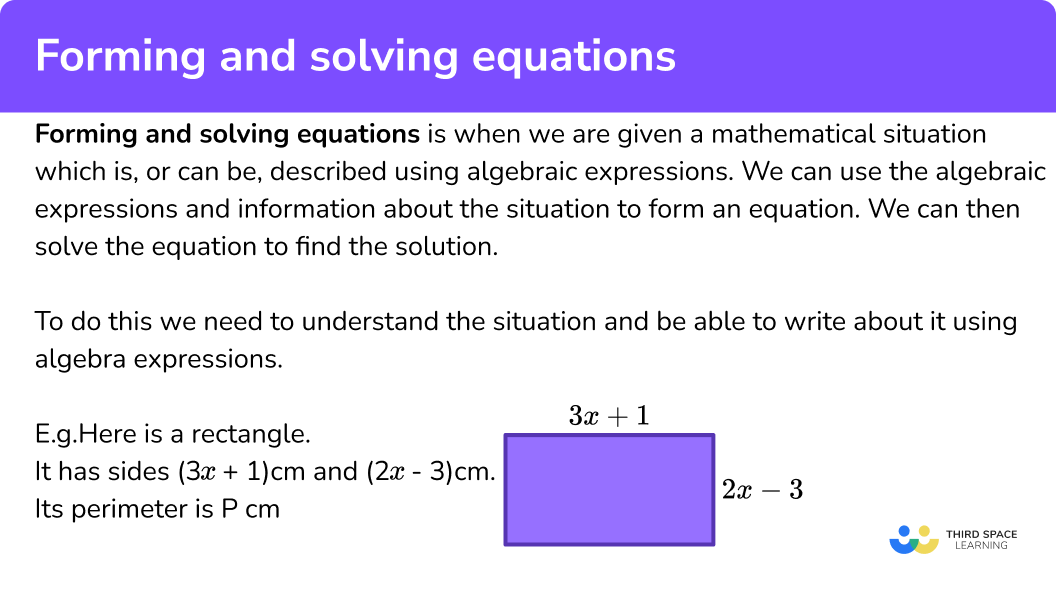### What is forming and solving equations?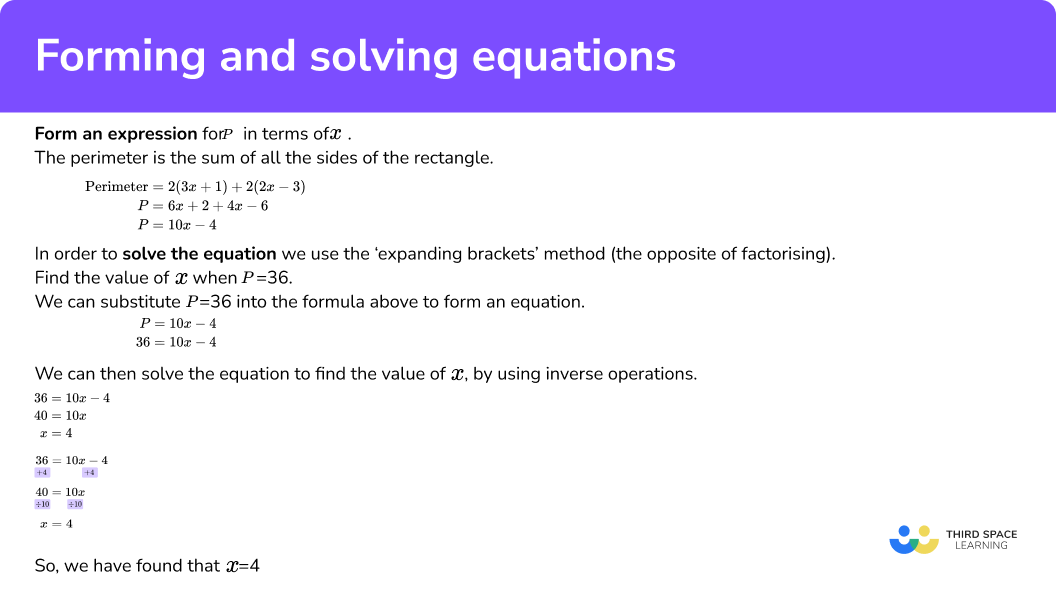### Forming an expression

We can take information from a question and use it to formulate algebraic expressions.

For example, here is a diagram of a regular pentagon.

We can form an expression for the perimeter of the pentagon.

\text{Perimeter }=5(x+4)

An expression is a number of algebraic terms combined by adding, subtracting, multiplying and dividing whereas an equation sets two expressions equal to each other.

### Form an equation

We can then use expressions to set up equations which can then be solved.

For example, here is a diagram with parallel lines.

We can form an equation from the diagram as corresponding angles in parallel lines are equal.

3x-15=2x+10

## How to form and solve equations

In order to form and solve equations:

1. Write the situation using algebra.
2. Use the information to make the algebraic equation.
3. Solve the equation.

### Explain how to form and solve equations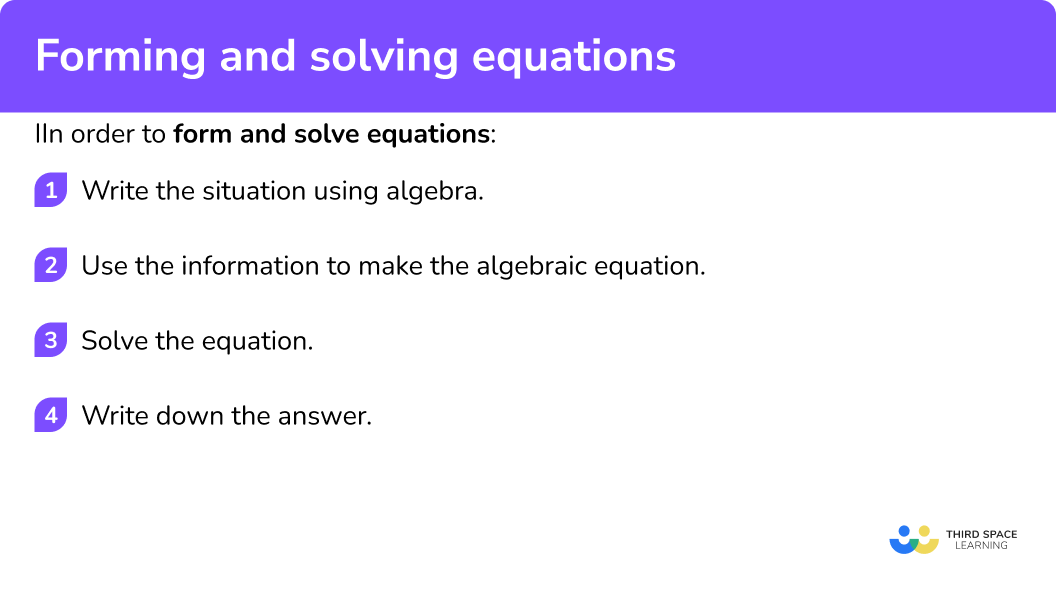## Forming and solving equations examples

### Example 1: ages

Abi is x years old.

Bob is twice as old as Abi.

Cam is 3 years older than Bob.

The total of their ages is 58.

Form an equation and solve to find x.

1. Write the situation using algebra.

The situation has three ages. We know that Abi is x years old.

We need to write expressions for the ages of Bob and Cam.

Abi is x years old.

Bob is twice as old as Abi. Therefore Bob is 2x years old.

Cam is 3 years older than Bob therefore Cam is (2x+3) years old.

We are given information about the total of their ages, so we need to add the ages together.

\begin{aligned} \text{Total}&=x+2x+(2x+3)\\\\ \text{Total}&=5x+3 \end{aligned}

2Use the information to make the algebraic equation.

We are given the information that the total of the ages is 58. So, instead of “total” we can write “58”.

58=5x+3

3Solve the equation.

The value of x is 11.

Note: We can check our answer by substituting back into the original situation.

Abi is x years old and so Abi is 11 years old.

Bob is twice as old as Abi and so Bob is 22 years old.

Cam is 3 years older than Bob and so Cam is 25 years old.

11+22+25=58.

The total is correct and therefore we know our answer is correct.

### Example 2: perimeter

This is a regular pentagon.

Each side is (3x-4)cm.

The perimeter of the pentagon is 70 \ cm.

Form an equation and solve to find x.

The situation is about the perimeter of a regular pentagon. Since it is regular, all the sides of the shape are the same. The perimeter is,

\begin{aligned} \text{Perimeter}&= 5(3x-4) \\\\ \text{Perimeter}&= 15x-20 \end{aligned}

We are given the information that the perimeter is 70. So, instead of “perimeter” we can write “70”.

70=15x-20

The value of x is 6.

### Example 3: angles

Form an equation and solve to find x.

The situation is about the parallel lines and angles. We are given 2 angles, 6x-20 and 4x+15.

The two angles we are given are equal to each other since they are alternate angles. Therefore we can write,

6x-20=4x+15.

The value of x is 17.5.

### Example 4: probability

In a box there are only blue counters, green counters, red counters and yellow counters.

Dana is going to take at random a counter from the box.

The probability of taking a red counter is twice the probability of taking a yellow counter.

Form an equation and solve to find the probability that Dana picks a yellow counter.

The situation is about the probability of picking counters. We have not been given the probabilities for picking a red counter or a yellow counter. But we have been given some information.

We can let the probability of picking a yellow counter be x. Then the probability of picking a red counter will be 2x.

We need to use the fact that the sum of probabilities is 1.

0.15+0.25+2x+x=1

The left hand side of the equation can be simplified by collecting the like terms.

0.4+3x=1

The value of x is 0.2. But the questions asks for the probability of picking a yellow counter which is 2x.

Therefore the probability of picking a yellow counter is

2\times 0.2=0.4.

The probability of picking a yellow counter is 0.4.

### Example 5: averages

Charlie is 174 \ cm tall. Erica is x \ cm shorter than Charlie.

The mean of their heights is 169 \ cm.

Form an equation and use it to find how tall Erica is.

The situation is about the mean of two heights. Erica’s height is (174-x)cm. To find the mean we find the total of the values and divide by the number of values.

\text{Mean}=\frac{174+(174-x)}{2}

The right hand side can be simplified giving

\text{Mean}=\frac{348-x}{2} .

We can use the information that the mean is 169 and replace “mean” with this value.

169=\frac{348-x}{2}

The value of x is 10. But the questions asks for Erica’s height which is 174-x.

Therefore Erica’s height is

174-10=164.

Erica’s height is 164 \ cm.

The width of a rectangle is x \ cm. Its length is 2 \ cm longer than its width.

The area of the rectangle is 48 \ cm^{2}.

Form an equation and solve it to find the width of the rectangle.

The situation is about the area of a rectangle. The area of a rectangle is found by multiplying the two adjacent sides.

\text{Area}=x(x+2).

We can use the information that the area is 48 and replace “area” with this value.

48=x(x+2)

We can solve the equation. First we expand the brackets,

\begin{aligned} 48&=x(x+2) \\\\ 48&= x^2+2x \end{aligned}

This is a quadratic equation. We rearrange the equation so that one side is equal to 0 and the expression can then be factorised and solved.

The value of x is -8 or 6. Since the question asks for the width of a rectangle, we can ignore the negative answer as a length cannot be negative.

The width of the rectangle is therefore 6 \ cm.

### Common misconceptions

• Answers can be integers, fractions or decimals

Answers to equations can be integers, fractions or decimals. You can always check if your answer is correct by substituting the answer back into the original situation.

• Algebraic expressions should be simplified

Once you have written the situation using algebra, the algebra should be simplified. This may mean expanding brackets and simplifying.

You may have solved the equation to find x, but the questions may want something slightly different. For example the length of the longest side.

### Practice forming and solving equationsquestions

1. Form an equation and solve to find Raj’s age.

Raj is x years old.

Sam is twice as old as Raj.

Tina is 2 years younger than Raj.

The total of their ages is 58 years.

4x+2=58 \ \text{and} \ x=154x-2=58 \ \text{and} \ x=164x-2=58 \ \text{and} \ x=155x-2=58 \ \text{and} \ x=12The ages are x, \ 2x and (x-2).

\begin{aligned} \text{Total}&=x+2x+(x-2) \\\\ \text{Total}&= 4x-2\\\\ 58&=4x-2 \end{aligned}

So the equation is 4x-2=58.

This equation can be solved,

\begin{aligned} 4x-2&=58 \\\\ 4x&= 60\\\\ x&=15 \end{aligned}

So the solution is x=15.

2. The perimeter of this regular hexagon is 108 \ cm. Form an equation and solve to find x.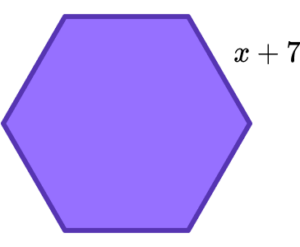6x+42=108 \ \text{and} \ x=116x+42=108 \ \text{and} \ x=125x+7=108 \ \text{and} \ x=14.66x+7=108 \ \text{and} \ x=16.8The shape is a regular hexagon. To find its perimeter we can multiply the side length by 6.

\begin{aligned} \text{Perimeter}&=6(x+7) \\\\ \text{Perimeter}&=6x+42\\\\ 108&= 6x+42 \end{aligned}

So the equation is 6x+42=108.

This equation can be solved,

\begin{aligned} 6x+42&= 108\\\\ 6x&=66\\\\ x&=11 \end{aligned}

So the solution is x=11.

3. Form an equation and solve to find x.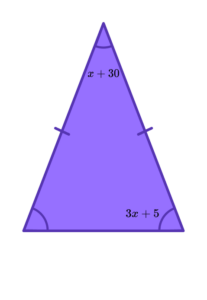4x+35=180 \ \text{and} \ x=207x+40=180 \ \text{and} \ x=204x+35=180 \ \text{and} \ x=20.77x+40=180 \ \text{and} \ x=15The shape is an isosceles triangle so the angles are (x+30), \ (3x+5) and (3x+5). The angles in a triangle add up to 180^{o}.

\begin{aligned} \text{Total}&=(x+30)+(3x+5)+(3x+5) \\\\ \text{Total}&=7x+40\\\\ 180&=7x+40 \end{aligned}

So the equation is 7x+40=180.

This equation can be solved,

\begin{aligned} 7x+40&= 180\\\\ 7x&=140\\\\ x&=20 \end{aligned}

So the solution is x=20.

4. Here are the probabilities for picking different coloured counters from a bag. The probability of picking a white counter at random is x. The probability of picking a black counter is three times the probability of picking a white counter.

Form an equation and solve to find the probability of picking a white counter.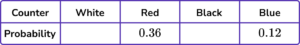4x+0.48=1 \ \text{and} \ x=0.262x+0.48=1 \ \text{and} \ x=0.134x+0.48=1 \ \text{and} \ x=0.132x+0.48=1 \ \text{and} \ x=0.13This missing probabilities will be,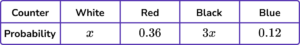\begin{aligned} \text{Total of probabilities}&=x+0.36+3x+0.12\\\\ \text{Total of probabilities}&= 4x+0.48\\\\ 1&=4x+0.48 \end{aligned}

So the equation is 4x+0.48=1.

This equation can be solved,

\begin{aligned} 4x+0.48&= 1\\\\ 4x&=0.52\\\\ x&=0.13 \end{aligned}

So the solution is x=0.13.

5. There are three bags of sweets.
In the first bag there are n sweets.
In the second bag there are (n+5) sweets.
In the third bag there are (2n-3) sweets.

The mean number of sweets in the bags is 22.

Form an equation and solve to find n.

\frac{4n+2}{3}=22 \ \text{and} \ n=16\frac{3n+2}{4}=22 \ \text{and} \ n=15\frac{3n+2}{4}=22 \ \text{and} \ n=16\frac{4n+2}{3}=22 \ \text{and} \ n=15The total of the three bags is,

n+(n+5)+(2n-3)=4n+2.

\begin{aligned} \text{Mean}&=\frac{\text{Total}}{3}\\\\ \text{Mean}&=\frac{4n+2}{3}\\\\ 22&=\frac{4n+2}{3} \end{aligned}

So the equation is \frac{4n+2}{3}=22.

This equation can be solved,

\begin{aligned} \frac{4n+2}{3}&=22\\\\ 4n+2&=66\\\\ 4n&=64\\\\ n&=16 \end{aligned}

So the solution is n=16.

6.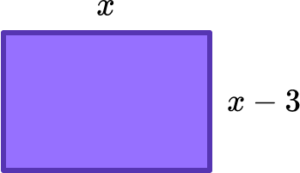The area of the rectangle is 54 \ cm^{2}.

Form an equation and solve to find x.

x(x-3)=54 \ \text{and} \ x=6x(x-3)=54 \ \text{and} \ x=92x+2(x-3)=54 \ \text{and} \ x=15x+(x-3)=54 \ \text{and} \ x=28.5The area of the rectangle can be found by multiplying the two sides together,

\begin{aligned} \text{Area}&=x\times (x-3)\\\\ \text{Area}&=x(x-3)\\\\ 54&=x(x-3) \end{aligned}

So the equation is x(x-3)=54.

This equation can be solved by expanding the brackets. The equation is a quadratic equation.

\begin{aligned} x(x-3)&=54\\\\ x^2-3x&=54\\\\ x^2-3x-54&=0\\\\ (x+6)(x-9)&=0\\\\ \text {therefore} \ x=-6 & \;\; \text{or} \;\; x=9 \end{aligned}

Since the question is about the sides of a rectangle, the solution is x=9 because it must be positive.

### Forming and solving equations GCSE questions

1. Andrew has x sweets.

Bob has 7 more sweets than Andrew.

Charlie has twice as many sweets as Bob.

The total number of sweets is 89.

(a) Write an expression for the number of sweets that Bob has.

(b) Write an expression for the number of sweets that Charlie has.

(c) Form an equation about the total number of sweets and solve it to find x.

(4 marks)

(a)

x+7

(1)

(b)

2(x+7) or 2x+14

(1)

(c)

4x+21=89

(1)

x=17

(1)

2. In the diagram all measurements are in cm.

ABC is an isosceles triangle.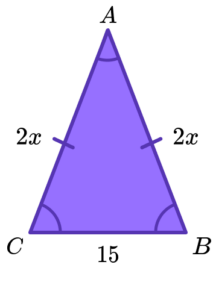\begin{aligned} &AB=2x \\ &BC=15 \\ &AC=2x \end{aligned}

(a) Find an expression, in terms of x, for the perimeter of the triangle.

(b) The perimeter of the triangle is 65 \ cm.

Find the value of x.

(4 marks)

(a)

2x+2x+15

(1)

4x+15

(1)

(b)

4x+15=65

(1)

x=12.5

(1)

3. Here is a diagram showing two angles.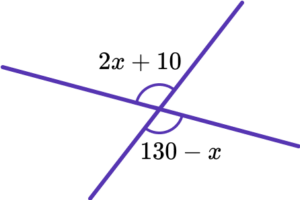(a) Explain why 2x+10=130-x.

(b) Find the size of the angle 2x+10.

(4 marks)

(a)

Vertically opposite angles are equal.

(1)

(b)

3x=120

(1)

x=40

(1)

2x+10=90

(1)

## Learning checklist

You have now learned how to:

• Use expressions to form an equation
• Form equations from a variety of situations

## Still stuck?

Prepare your KS4 students for maths GCSEs success with Third Space Learning. Weekly online one to one GCSE maths revision lessons delivered by expert maths tutors.

Find out more about our GCSE maths revision programme.

x

#### FREE GCSE Maths Practice Papers - 2022 Topics

Practice paper packs based on the advanced information for the Summer 2022 exam series from Edexcel, AQA and OCR.

Designed to help your GCSE students revise some of the topics that will come up in the Summer exams.